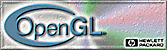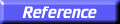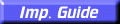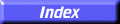# glMultMatrix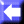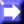glMultMatrixd, glMultMatrixf: multiply the current matrix with the specified matrix.

C Specification | Parameters | Description | Examples | Notes | Errors | Associated Gets | See Also

##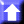C Specification

```void glMultMatrixd(
const GLdouble *m)
void glMultMatrixf(
const GLfloat  *m)
```

##Parameters

m
Points to 16 consecutive values that are used as the elements of a 4
4 column-major matrix.

##Description

glMultMatrix multiplies the current matrix with the one specified using m, and replaces the current matrix with the product.

The current matrix is determined by the current matrix mode (see glMatrixMode). It is either the projection matrix, modelview matrix, or the texture matrix.

##Examples

If the current matrix is C, and the coordinates to be transformed are, v = (v, v, v, v. Then the current transformation is Cv, or

 C C C C C C C C C C C C C C C Cv v v v

Calling glMultMatrix with an argument of m = m, m, . . ., m replaces the current transformation with (Cm )v, or

 C C C C C C C C C C C C C C C Cm m m m m m m m m m m m m m m mv v v v

Where "" denotes matrix multiplication, and v is represented as a 41 matrix.

##Notes

While the elements of the matrix may be specified with single or double precision, the GL may store or operate on these values in less than single precision.

In many computer languages, 44 arrays are represented in row-major order. The transformations just described represent these matrices in column-major order. The order of the multiplication is important. For example, if the current transformation is a rotation, and glMultMatrix is called with a translation matrix, the translation is done directly on the coordinates to be transformed, while the rotation is done on the results of that translation.

##Errors

• GL_INVALID_OPERATION is generated if glMultMatrix is executed between the execution of glBegin and the corresponding execution of glEnd.

##Associated Gets

glGet with argument GL_MATRIX_MODE
glGet with argument GL_MODELVIEW_MATRIX
glGet with argument GL_PROJECTION_MATRIX
glGet with argument GL_TEXTURE_MATRIX